6390瀏覽
Script for automatic trading (Alerts). This is the second part of the previous RePanoCHa script but with Take Profit.

It Use 6 indicators + volume:

-JURIK MOVING AVERAGE
-RANGE FILTER (DONOVAN WALL)
-PARABOLIC SAR
-RSI + VOLUME WEIGHTED (LAZYBEAR)
-MACD
-VOLUME

To close the position you can choose between Take Profit only, Trailing Stop only or both at the same time.

Example of bot syntax: (alerts once per bar)

LONG | e=BINANCEFUTURES a=ACCOUNT s=BTCUSDT c=order | delay=1 | e=BINANCEFUTURES a=ACCOUNT s=BTCUSDT c=position b=short t=market ro=1 | delay=1 | e=BINANCEFUTURES a=ACCOUNT s=BTCUSDT b=long q=100% t=market | delay=1 | e=BINANCEFUTURES a=ACCOUNT s=BTCUSDT c=position b=long p=0.7% q=50% t=post ro=1

SHORT | e=BINANCEFUTURES a=ACCOUNT s=BTCUSDT c=order | delay=1 | e=BINANCEFUTURES a=ACCOUNT s=BTCUSDT c=position b=long t=market ro=1 | delay=1 | e=BINANCEFUTURES a=ACCOUNT s=BTCUSDT b=short q=100% t=market | delay=1 | e=BINANCEFUTURES a=ACCOUNT s=BTCUSDT c=position b=short p=-0.7% q=50% t=post ro=1

LONG TP | e=BINANCEFUTURES a=ACCOUNT s=BTCUSDT c=position q=50% t=market ro=1 (if you set the TP from entry this alert is not necessary)

SHORT TP | e=BINANCEFUTURES a=ACCOUNT s=BTCUSDT c=position q=50% t=market ro=1 (if you set the TP from entry this alert is not necessary)

LONG TS | e=BINANCEFUTURES a=ACCOUNT s=BTCUSDT c=position q=10% t=market // (alerts once per minute)

SHORT TS | e=BINANCEFUTURES a=ACCOUNT s=BTCUSDT c=position q=10% t=market // (alerts once per minute)

XLONG/STOP-LOSS | e=BINANCEFUTURES a=ACCOUNT s=BTCUSDT c=order | delay=1 | e=BINANCEFUTURES a=ACCOUNT s=BTCUSDT c=position b=long t=market ro=1

XSHORT/STOP-LOSS | e=BINANCEFUTURES a=ACCOUNT s=BTCUSDT c=order | delay=1 | e=BINANCEFUTURES a=ACCOUNT s=BTCUSDT c=position b=short t=market ro=1

## 評論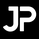Hey your script looks really good. I mainly use only the short and long condtions. But these work great.
I have recently enabled the trailing stop and stop loss function and had no problems so far.
However, since yesterday evening a problem occurred. Your script opens a trade and does not close it anymore. Both the TS and SL are not executed.
You can see this at my charti in the green box (18 April at 12pm (UTC)):

The error occurs with the following settings on the chart BTCUSD Perpetual Contract 1h BYBIT on 18 April at 12pm (UTC).

I use the default settings.
I only change the following things.

- Change exit strategy from BOTH to TRAILING STOP
- ACTIVATE STOP LOSS
- Set the STOP LOSS to 2%

Do you have an idea what could be the reason for this?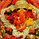Why its saying the indicator can re paint warning while setting alert. does it re paint?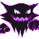So for example?
e=BINANCEFUTURES a=ACCOUNT s=BTCUSDT b=long q=100% t=market
One for TP:
e=BINANCEFUTURES a=ACCOUNT s=BTCUSDT c=position q=50% t=market ro=1
and one for TS:
e=BINANCEFUTURES a=ACCOUNT s=BTCUSDT c=position q=10% t=market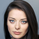You have a mistake here
----
LONG | e=BINANCEFUTURES a=ACCOUNT s=BTCUSDT c=order | delay=1 | e=BINANCEFUTURES a=ACCOUNT s=BTCUSDT c=position b=short t=market ro=1 | delay=1 | e=BINANCEFUTURES a=ACCOUNT s=BTCUSDT b=long q=100% t=market | delay=1 | e=BINANCEFUTURES a=ACCOUNT s=BTCUSDT c=position b=long p=0.7% q=50% t=post ro=1

SHORT | e=BINANCEFUTURES a=ACCOUNT s=BTCUSDT c=order | delay=1 | e=BINANCEFUTURES a=ACCOUNT s=BTCUSDT c=position b=long t=market ro=1 | delay=1 | e=BINANCEFUTURES a=ACCOUNT s=BTCUSDT b=short q=100% t=market | delay=1 | e=BINANCEFUTURES a=ACCOUNT s=BTCUSDT c=position b=short p=-0.7% q=50% t=post ro=1
---

in last command

---
e=BINANCEFUTURES a=ACCOUNT s=BTCUSDT c=position b=long p=0.7% q=50% t=post ro=1
---

it is TP1 right? Then "b" need to be "short" for LONG position?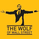Which option will we choose for unconfirmed signal alarm options?